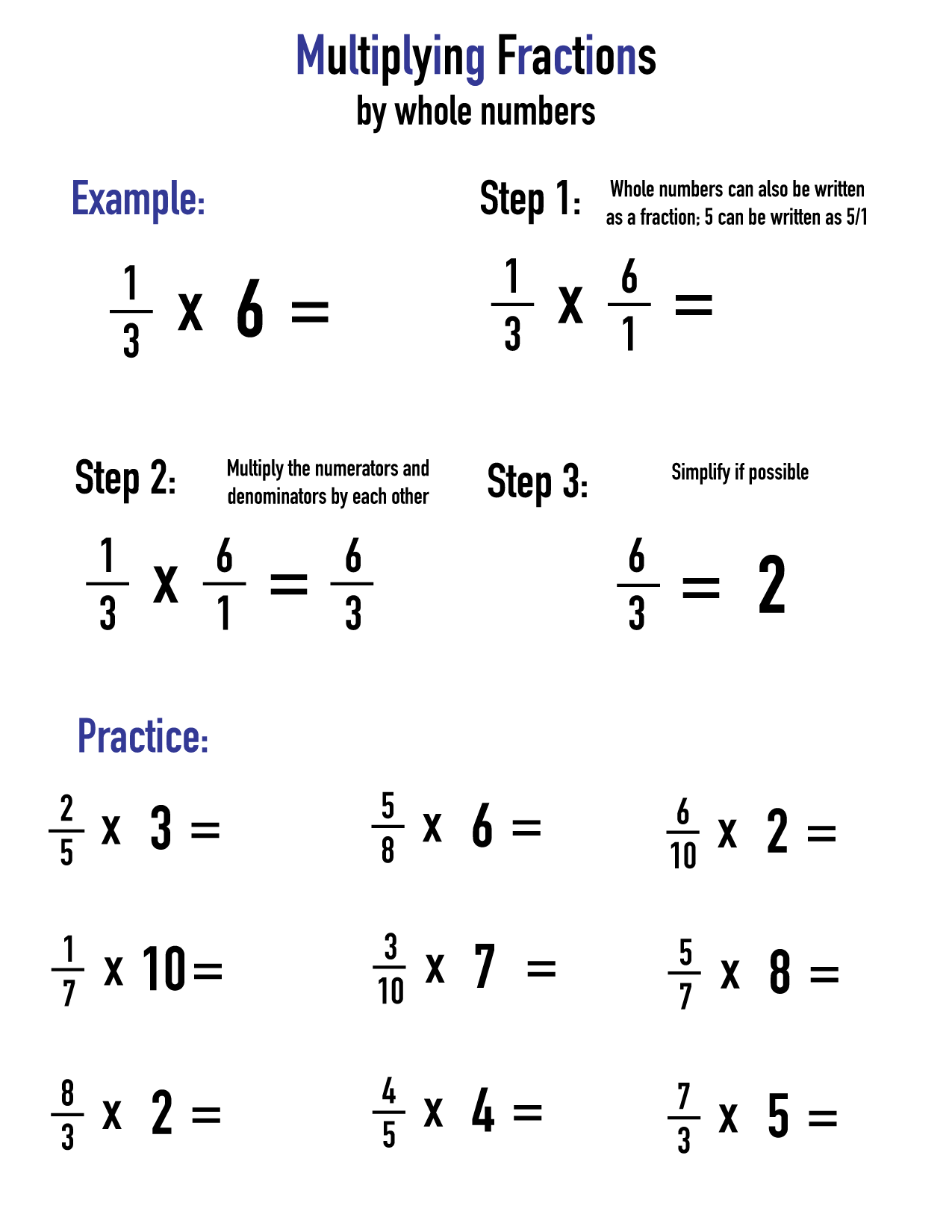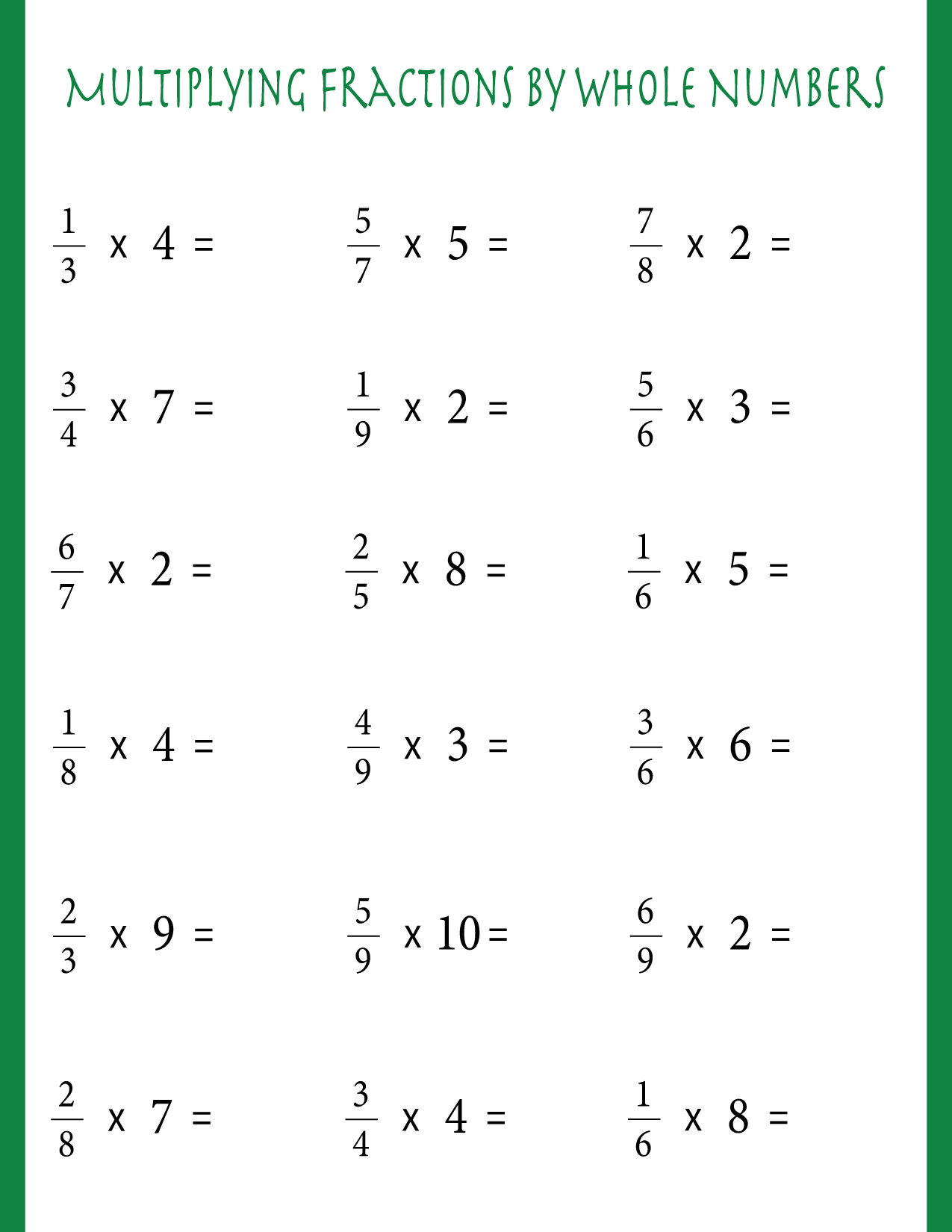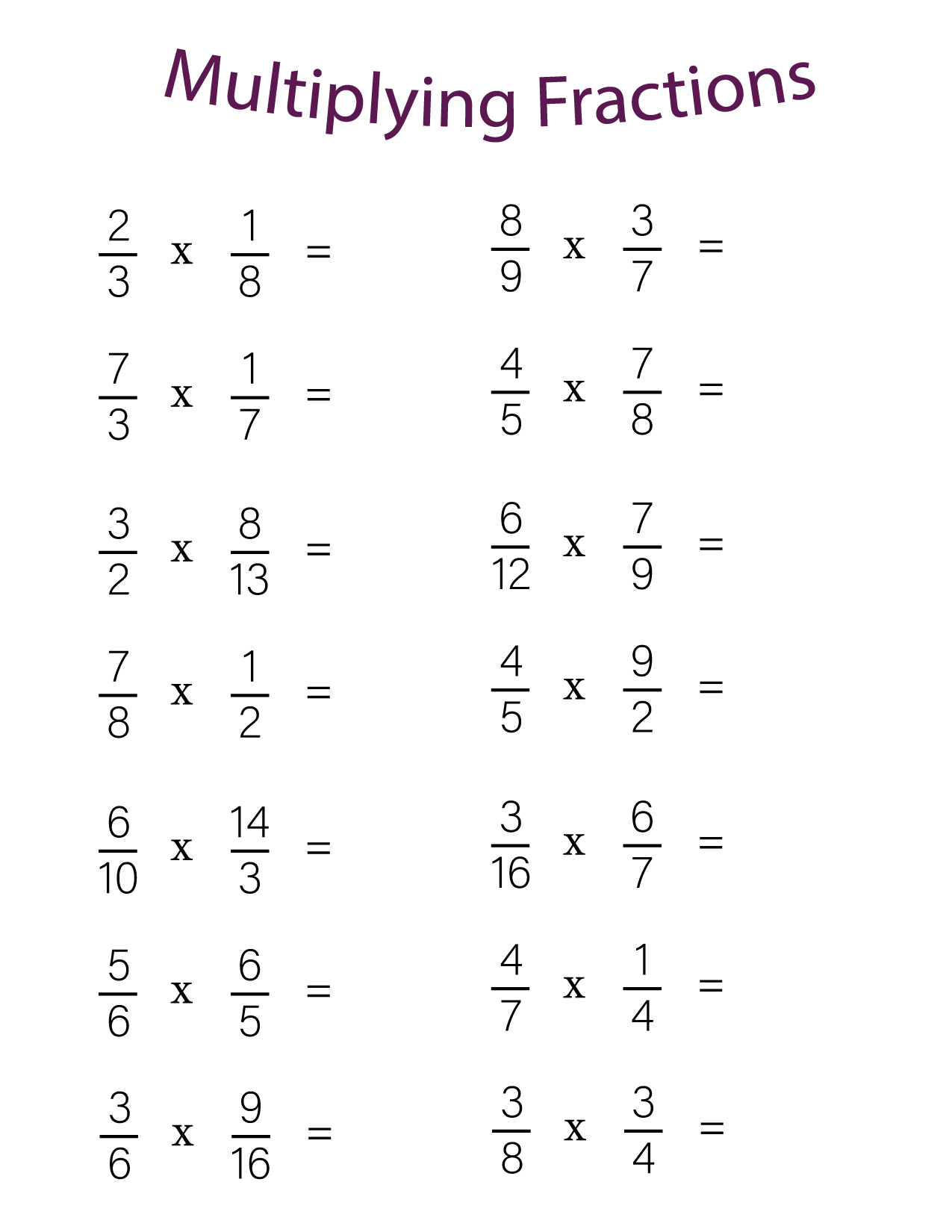Multiplying fractions is not that hard once you get used to it. In this post, we will get kids used to multiplying fractions by teaching them how it works and then giving them worksheets to practice!

Do you need more fraction multiplication worksheets? If so, check out our post here. If you need to work more on adding and subtracting fractions, we have those for you too! Printerfriend.Ly has so many math worksheets for you to look at – we even have practice sets for different grades of elementary school. Have a look around and you’ll probably find what you need!

## Multiplying fractions with instructions

Our first sheet shows how to multiply fractions by whole numbers, and gives some practice problems as well. If you need other tips on multiplying fractions, check out this post, which has examples as well.## Multiplying fractions by whole numbers

Our next worksheet continues multiplying fractions by whole numbers. Remember that a whole number can be turned into a fraction by putting it over the denominator of ‘1’!## Multiplying fractions #1

When you’re not multiplying fractions by a whole number, the same methodology applies. Simply multiply the numerators and the denominators by each other and then simplify. If you need advice on how to do this, check out this post.